#### Howdy, Stranger!

We are about to switch to a new forum software. Until then we have removed the registration on this forum.

# Image Texture Mode strange Mapping 360

edited May 2017

I tested this code with other frameworks/engines It works fine.

In processing with different images/videos etc. you can see a line going through the screen

I guess, it's the default texture mode. Maybe a user already got a workaround

Any help is much appreciated.

Copy +Paste press Play

``````//Vertex
String[] vertSource={"#if __VERSION__ >= 150" //check backward compatible /mobile
, "#define attribute in"
, "#define varying out"
, "#endif"
, "#ifdef GL_ES"
, "precision mediump float;"
, "precision mediump int;"
, "#endif"

//attribute
, "in vec4 position;"
//uniforms
, "uniform mat3 Ry;"
, "uniform float pitch;"
//varying
, "out vec3 refDir;"

, "void main() {"
, "gl_Position = vec4(position.xy,0, 1.);"

//apply Rotation Matix
, "refDir = Ry*vec4(position.xy,1.,0).xyz;"
, "}"
};

//Fragment
String[] fragSource={"#if __VERSION__ >= 150" //check backward compatible /mobile
, "#define attribute in"
, "#define varying out"
, "#define texture(a,b) texture2D(a,b)"
, "#endif"
, "#ifdef GL_ES"
, "precision mediump float;"
, "precision mediump int;"
, "#endif"

//attribute
, "in vec3 refDir;"
//uniforms
, "uniform sampler2D envmap;"
//varying
, "out vec4 fragColor;"

, "#define PI 3.14159273"

, "void main () {"

//map texture to sphere: <a href="https://github.com/mrdoob/three.js/issues/1621" target="_blank" rel="nofollow">https://github.com/mrdoob/three.js/issues/1621</a>;
, "float lat = atan(refDir.z,refDir.x);"
, "float lon = acos(refDir.y/length(refDir));"
, "fragColor = texture(envmap,vec2(.5+lat/(2.0*PI),lon/PI));"
, "}"
};

PImage img;
PMatrix3D rotY = new PMatrix3D();

void setup() {
//setup scene
size(640, 360, P3D);

//https://www.flickr.com/search/?l=commderiv&q=equirectangular
String https="https://"; //processing forum resolve links automatically - workaround to avoid it
}
void draw() {

//map mouse to a range form - to
float p=map(mouseX, 0, width, HALF_PI, -PI);

//uncomment for 360 rotation
//p=(float)millis()*.001f;

//rotation to mouse
float s=sin(p), c=cos(p);

//uniform Rotation Matrix

//reset to NULL
rotY.reset();
//Rotation (pitch) "http://planning.cs.uiuc.edu/node102.html/
rotY.apply(
c, 0, s, 0,
0, 1, 0, 0,
-s, 0, c, 0,
0, 0, 0, 1);

//clear
background(0);

//uniform texture image

//apply filter to scene
}
``````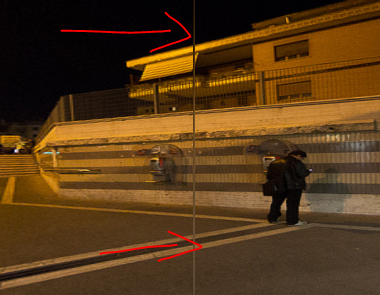Tagged:

• edited May 2017 Answer ✓

How i suspected the error occurs in the wrorg texture filtering mode, switch to

((PGraphicsOpenGL)g).textureSampling(2); //nearest

fixed it.

``````PShape s;PShader bgShader, bunnyShader;
PMatrix3D rotY=new PMatrix3D();
void setup() {
size(640,360,P3D);

noStroke();
noLights();
noSmooth();
camera(); //empty Object ?
hint(DISABLE_OPTIMIZED_STROKE); //comment this out at hi-res

new String[]{"\n in vec4 position; "
+ "uniform mat4 Ry,modelview;"
+ "out vec4 refDir;"
+ "void main() {"
+ "gl_Position = vec4(position.xy-1.,0, 1.);"
+ "refDir = modelview*Ry*vec4(position.xy-1,1.,0);"
+ "}"
}, new String[] {"\n in vec4 refDir;"
+ "uniform sampler2D envmap;"
+ "out vec4 fragColor;"
+ "const float PI ="+(double)PI+";"
+ "void main () {"
+ "fragColor = texture(envmap,"
+                "vec2(.5+atan(refDir.z,refDir.x)/(2.0*PI),"
+                        "acos(refDir.y/length(refDir))/PI));"
+ "}"
}){
((PGraphicsOpenGL)g).textureSampling(2);
this.set("modelview",((PGraphicsOpenGL) g).modelview);
return this;
}
}.run();
//
new String[]{"\n in vec3 position,normal;"
+ "uniform mat4 modelviewInv,modelview,projection;"
+ "uniform float time;"
+ "out vec3 refDir;"
+ "void main () {"
+ "vec4 camPos = modelview*vec4(position, 1);"
+ "vec3 eye = normalize(position.xyz-modelviewInv.xyz/modelviewInv.w);"
+ "refDir = reflect(eye, normal);"
+ "camPos.z*=clamp(sin(time)*.5+.5,0,0.5);"
+ "gl_Position = projection*camPos;"
+ "}"
},new String[]{"\n in vec3 refDir;"
+ "uniform sampler2D envmap;"
+ "out vec4 fragColor;"
+ "const float PI ="+(double)PI+";"
+ "void main () {"
+ "fragColor = texture(envmap,"
+                "vec2(.5+atan(refDir.z,refDir.x)/(2.0*PI),"
+                        "acos(refDir.y/length(refDir))/PI));"
+"}"
}){
this.set("modelviewInv",((PGraphicsOpenGL)g).modelviewInv);
this.set("modelview",((PGraphicsOpenGL)g).modelview);
this.set("projection",((PGraphicsOpenGL)g).projection);
return this;
}
}.run();
}void draw(){

background(0);// just to be nice

final float t=millis()*.001f;

rotY.reset();
rotY.apply(cos(t), 0, sin(t), 0, 0, 1, 0, 0, -sin(t), 0, cos(t), 0, 0, 0, 0, 1);

rect(0, 0, width, height);

translate(width/2, height/2+50);rotateZ(PI);rotateY(t);scale(50);shape(s);
resetShader(); // just to be nice

if(keyPressed||mousePressed)exit();
}
``````
• hi nabr, found this by following a link someone posted here:

got some errormessages about non-varying refDir and fragColor. after defining these as varying variables, it seems to work to fine for me, for now

• Hello @HBo

• pc win7 64 bit processing 3.0.1

one question: when I comment hint(DISABLE_OPTIMIZED_STROKE), there appears a seam in the reflection -->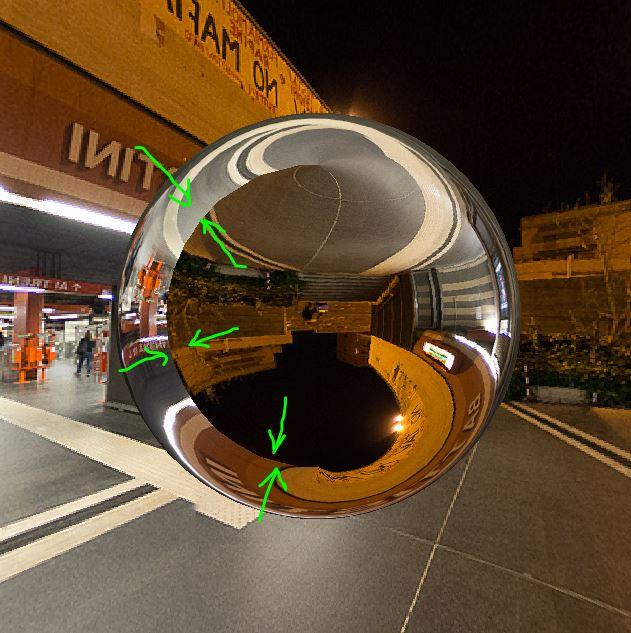@ everybody do you know how to fix it? (please don't pay attention to the wrong relation of environment - reflection. i only need to get rid of that seam on the sphere)

• @HBo: Please share your source. So i can catch up. I curretly bussy with my own stuff. when i setup the processing with a sphere already 10mins pass. And it's not garanted that i fix the problem.

So i can easily copy paste and start with debug. (You can also provide a zip file, would be also nice.)

• edited May 2017

@HBo This is not 100%, but from my experience

you look INSIDE the sphere. AND i don't know how to fix it. Something wrong with the normals. they should be flipped. And pointing always towards the camera

For Testing:

``````PShape s;PShader bgShader, bunnyShader;
PMatrix3D rotY=new PMatrix3D();
void setup() {
size(640,360,P3D);

noStroke();
//i commented the most stuff out.
//noLights();
//noSmooth();
//camera(); //empty Object ?
// hint(DISABLE_OPTIMIZED_STROKE); //comment this out at hi-res

new String[]{"\n in vec4 position; "
+ "uniform mat4 Ry,modelview;"
+ "out vec4 refDir;"
+ "void main() {"

//prev version position.xy-1 becourse of double buffer in processing
+ "gl_Position = vec4(position.xy,0, 1.);"
+ "refDir = modelview*Ry*vec4(position.xy,1.,0);"
+ "}"
}, new String[] {"\n in vec4 refDir;"
+ "uniform sampler2D envmap;"
+ "out vec4 fragColor;"
+ "const float PI ="+(double)PI+";"
+ "void main () {"
+ "fragColor = texture(envmap,"
+                "vec2(.5+atan(refDir.z,refDir.x)/(2.0*PI),"
+                        "acos(refDir.y/length(refDir))/PI));"
+ "}"
}){
((PGraphicsOpenGL)g).textureSampling(2);
this.set("modelview",((PGraphicsOpenGL) g).modelview);
return this;
}
}.run();
//
new String[]{"\n in vec3 position,normal;"
+ "uniform mat4 modelviewInv,modelview,projection;"
//+ "uniform float time;"
+ "out vec3 refDir;"
+ "void main () {"
+ "vec4 camPos = modelview*vec4(position, 1);"
+ "vec3 eye = normalize(position.xyz-modelviewInv.xyz/modelviewInv.w);"
+ "refDir = reflect(eye, normal);"
//+ "camPos.z*=clamp(sin(time)*.5+.5,0,0.5);"
+ "gl_Position = projection*camPos;"
+ "}"
},new String[]{"\n in vec3 refDir;"
+ "uniform sampler2D envmap;"
+ "out vec4 fragColor;"
+ "const float PI ="+(double)PI+";"
+ "void main () {"
+ "fragColor = texture(envmap,"
+                "vec2(.5+atan(refDir.z,refDir.x)/(2.0*PI),"
+                        "acos(refDir.y/length(refDir))/PI));"
+"}"
}){
this.set("modelviewInv",((PGraphicsOpenGL)g).modelviewInv);
this.set("modelview",((PGraphicsOpenGL)g).modelview);
this.set("projection",((PGraphicsOpenGL)g).projection);
return this;
}
}.run();
}void draw(){
final float t=millis()*.001f;

rotY.reset();
rotY.apply(cos(t), 0, sin(t), 0, 0, 1, 0, 0, -sin(t), 0, cos(t), 0, 0, 0, 0, 1);

rect(0, 0, width, height);

background(127); //clear the background after the envmap is copied

translate(width/2, height/2);
sphere(140);

/*
stroke(4);
rotateY(1);
int count=0;
count=(mousePressed)?count+=t:count;
rotateZ(count);
box(140);
*/

//if(keyPressed||mousePressed)exit();
}
``````
• here's the code:

``````PShape s;PShader bgShader, bunnyShader;
PMatrix3D rotY=new PMatrix3D();
void setup() {
size(640,640,P3D);

noStroke();
noLights();
noSmooth();
camera(); //empty Object ?
//hint(DISABLE_OPTIMIZED_STROKE); //comment this out at hi-res

new String[]{"\n in vec4 position; "
+ "uniform mat4 Ry,modelview;"
+ "out varying vec4 refDir;"
+ "void main() {"
+ "gl_Position = vec4(position.xy-1.,0, 1.);"
+ "refDir = modelview*Ry*vec4(position.xy-1,1.,0);"
+ "}"
}, new String[] {"\n in vec4 refDir;"
+ "uniform sampler2D envmap;"
+ "out varying vec4 fragColor;"
+ "const float PI ="+(double)PI+";"
+ "void main () {"
+ "fragColor = texture(envmap,"
+                "vec2(.5+atan(refDir.z,refDir.x)/(2.0*PI),"
+                        "acos(refDir.y/length(refDir))/PI));"
+ "}"
}){
((PGraphicsOpenGL)g).textureSampling(2);
this.set("modelview",((PGraphicsOpenGL) g).modelview);
return this;
}
}.run();
//
new String[]{"\n in vec3 position,normal;"
+ "uniform mat4 modelviewInv,modelview,projection;"
+ "uniform float time;"
+ "out varying vec3 refDir;"
+ "void main () {"
+ "vec4 camPos = modelview*vec4(position, 1);"
+ "vec3 eye = normalize(position.xyz-modelviewInv.xyz/modelviewInv.w);"
+ "refDir = reflect(eye, normal);"
+ "camPos.z*=clamp(0.5,0,0.5);"
+ "gl_Position = projection*camPos;"
+ "}"
},new String[]{"\n in vec3 refDir;"
+ "uniform sampler2D envmap;"
+ "out varying vec4 fragColor;"
+ "const float PI ="+(double)PI+";"
+ "void main () {"
+ "fragColor = texture(envmap,"
+                "vec2(.5+atan(refDir.z,refDir.x)/(2.0*PI),"
+                        "acos(refDir.y/length(refDir))/PI));"
+"}"
}){
this.set("modelviewInv",((PGraphicsOpenGL)g).modelviewInv);
this.set("modelview",((PGraphicsOpenGL)g).modelview);
this.set("projection",((PGraphicsOpenGL)g).projection);
return this;
}
}.run();
}void draw(){

background(0);// just to be nice

final float t=millis()*.0001f;

rotY.reset();
rotY.apply(cos(t), 0, sin(t), 0, 0, 1, 0, 0, -sin(t), 0, cos(t), 0, 0, 0, 0, 1);

rect(0, 0, width, height);

translate(width/2, height/2);
//translate(0,100);
rotateZ(PI);

//scale(200);
//shape(s);
sphere(100);
resetShader(); // just to be nice

if(keyPressed||mousePressed)exit();
}
``````

when you replace the sphere with the bunny, you also can see easily see its seams (e.g. at the green areas):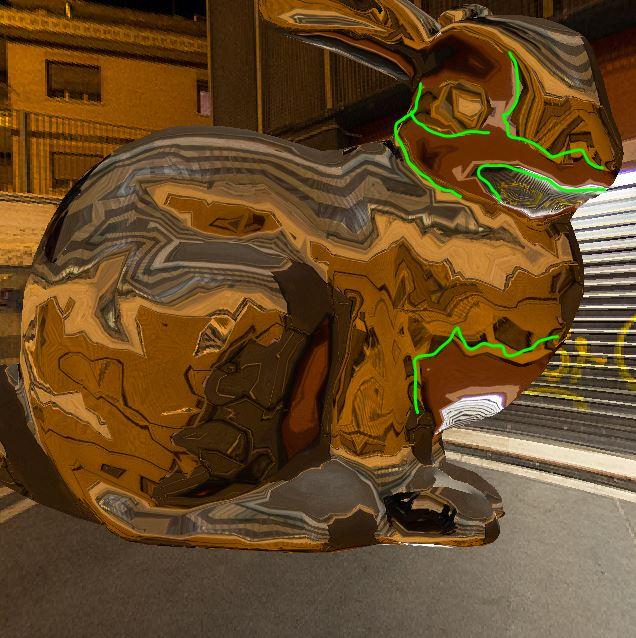• @HBo -- For that specific kind of projection you need a 360 panoramic image whose far left and right sides match up. Then there is no seam. For example, any of these images would work: https://www.google.com/search?q=360+panoramic+image&tbm=isch

Ah. I see that `envmap` is the correct type of image: I was wrong thinking that was the cause.• edited May 2017

@HBo i have to pass for today, but i will catch up soon. I think the default camara in processing have not the "right" settings, - that fits this task.

@jeremydouglass. No

Same shader code, accept for the cam.

https://cdn.rawgit.com/tolkanabroski/coding/master/regl/360/index.html

• edited May 2017

Here is a good example how to show the normals of a mesh. The outlines on the bunny comes from wrong normal direction.

i flip them here

`"vec3 eye = normalize(position.xyz-modelviewInv.xyz/modelviewInv.w);"`

so i have a custom situation and still the default camera.

• edited May 2017

@HBO i would love to fix it. But their no chance currently. Here is a good old but gold tutorial how camera works.

http://duriansoftware.com/joe/An-intro-to-modern-OpenGL.-Chapter-3:-3D-transformation-and-projection.html

first we shold pass a version i think this would solve the varying out problem `new String[]{"#version 150 \n"`

https://github.com/processing/processing/blob/master/core/src/processing/opengl/PGL.java#L1912

second the problem is that the normal attribe is predefined and wrong in this situation:

``````  + "in vec3 position,normal;"
``````

so you have to overwrite it: https://github.com/processing/processing/wiki/Advanced-OpenGL#processing-3x

``````shader.bind();
``````

@jeremydouglass

http://www.reindelsoftware.com/Documents/Mapping/Mapping.htmledit:

@HBo interessting effects !works only on a processing sphere

`+ "refDir = reflect(eye,vec3(normal.xy,1.-normal.z));"`

and flip the image `+ "refDir = reflect(-eye,-vec3(normal.xy,1.-normal.z));"`

• wow! thanks for your effort. I'll have a look at it tomorrow

• @nabr no seam on imported meshsphere created in rhino 3d: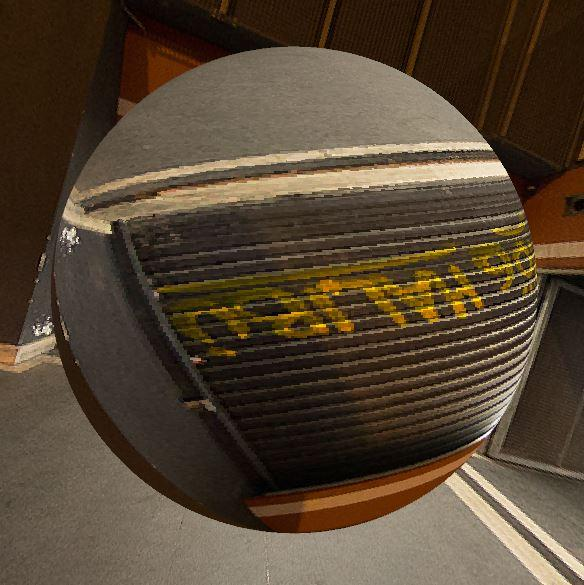• edited May 2017

@HBo

Okay i know now, its my mistake:

=>i have a spherical projection of a equirectangular image

=>i run the same code to map in back from equirectangular image to spherical projection

=>i should map it from spherical projection to spherical projection or better : from World Space To Spherical

``````+"vec3 n =  normalize(normal);"
+ "refDir = -n;"
``````

`+ "fragColor = texture(envmap,vec2( .5+asin(refDir.x/PI) ,.5+ asin(refDir.y/PI) )) ;"`

https://www.mvps.org/directx/articles/spheremap.htm

Full code comes tomorrow!

• @nabr yes, there should be made some changes with spherical projection. the reflection an a planar (very low-res) meshplane looks like this: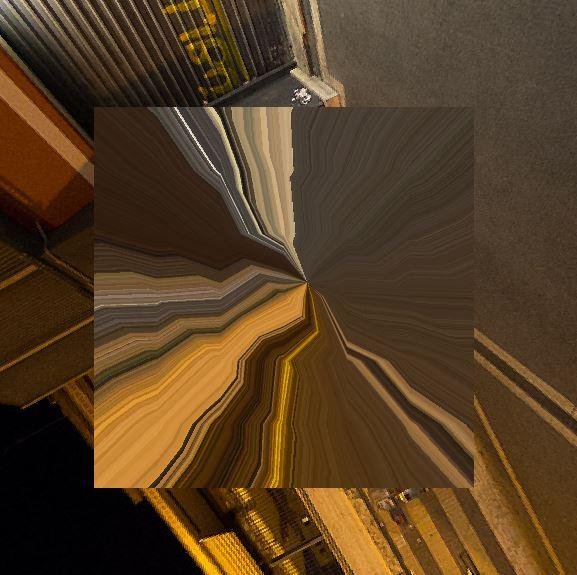i'm curious to see it...

• edited May 2017

@HBo

i dont really understand what is going on here i did a code clean up also read from forum post. this is what i got so far. I also out of time. Keep me posted with news:

``````//vertex pre multiplication done by processing

PMatrix3D rotY=new PMatrix3D();
PMatrix3D model = new PMatrix3D();
PMatrix3D modelview = new PMatrix3D();

float x =0;

void setup(){
size(856,480,P3D);

modelview=((PGraphicsOpenGL)g).modelview;

new String[]{"#version 150  \n"
+ "in vec4 position; "
+ "uniform mat4 Ry,view;"
+ "out vec4 refDir;"
+ "void main() {"
+ "gl_Position = vec4(position.xy,.0, 1.);"

//for reasons for taste i apply the rotation matrix first
+ "refDir =Ry*view*vec4(position.xy,1.,0);"
+ "}"
}, new String[] {"#version 150  \n"
+ "in vec4 refDir;"
+ "uniform sampler2D envmap;"
+ "out vec4 fragColor;"
+ "const float PI ="+(double)PI+";"
+ "void main () {"
//reindelsoftware.com/Documents/Mapping/Mapping.html
+ "fragColor = texture(envmap,"
+                "vec2(.5+atan(refDir.z,refDir.x)/(2.0*PI),"
+                        "acos(refDir.y/length(refDir))/PI));"
+ "}"
}){

//mode: linear
((PGraphicsOpenGL)g).textureSampling(2);

//somewhere else i run this minimal clear background
fill(x);
rect(x,x,width,height);
//draw grid
stroke(254);
while(x<width){
x+=20.01f;
line(x,0,x,height);
line(0,x,width,x);
} //x=0;

this.set("envmap",get() );

//for debug leave it here
//this.set("modelview",((PGraphicsOpenGL)g).modelview);

return this;
}
}.run();
new String[]{ "#version 150  \n"
+  "in vec3 position,normal;"
+ "uniform mat4 modelviewInv,view,projection;"
+ "uniform float time;"
+ "out vec3 refDir;"
+ "void main () {"
+ "vec4 camPos = view*vec4(position, 1);"
+ "vec3 eye = normalize(position.xyz-modelviewInv.xyz/modelviewInv.w);"
+ "refDir = reflect(eye, normal);"
// + "camPos.z*=clamp(sin(time)*.5+.5,0,0.5);"
+ "gl_Position = projection*camPos;"
+ "}"
},new String[]{"#version 150  \n"
+  "in vec3 refDir;"
+ "uniform sampler2D envmap;"
+ "out vec4 fragColor;"
+ "const float PI ="+(double)PI+";"
+ "void main () {"
/* + "fragColor = texture(envmap,"
+                "vec2(.5+atan(refDir.z,refDir.x)/(2.0*PI),"
+                        "acos(refDir.y/length(refDir))/PI));"*/
//mvps.org/directx/articles/spheremap.htm
+ "fragColor = texture(envmap,vec2( .5+asin(refDir.x/PI) ,.5+ asin(refDir.y/PI) ));"
+"}"
}){
this.set("modelviewInv",((PGraphicsOpenGL)g).modelviewInv);

//for debug leave it here
//this.set("modelview",   ((PGraphicsOpenGL)g).modelview);

this.set("projection",  ((PGraphicsOpenGL)g).projection);
return this;
}
}.run();

//with both options off/on you can see their some weird things happens

//for debug leave it here
//hint(DISABLE_OPTIMIZED_STROKE);

//for debug leave it here
//wireframe Mode
noStroke();
}
float t=0;
void draw(){

t=millis()*.0005f;

camera();
//rotate Camera
rotateX(sin(t));rotateZ(cos(t)*.5+.5);

//load the Identity matrix first PMatrix3D() returns the Identity matrix
//is far as i understand it.
//reset the stack
rotY.reset();
rotY.apply(cos(t), 0, sin(t), 0, 0, 1, 0, 0, -sin(t), 0, cos(t), 0, 0, 0, 0, 1);

//set time

//no depth testing

//for debug leave it here
//background(0);

rect(0, 0, width, height);

translate(width/2, height/2, 0);
sphere(120);

//after we apply the transformation to the sphere we update the matrix
//and going back to the begining of the draw loop ->camera

//load the modelview from stack first then update it

//reset the stack
model.reset();
model.apply(modelview.m00, modelview.m10, modelview.m20, modelview.m30,
modelview.m01, modelview.m11, modelview.m21, modelview.m31,
modelview.m02, modelview.m12, modelview.m22, modelview.m32,
modelview.m03, modelview.m13, modelview.m23, modelview.m33);

//for debug leave it here
//if(keyPressed||mousePressed)exit();
}
``````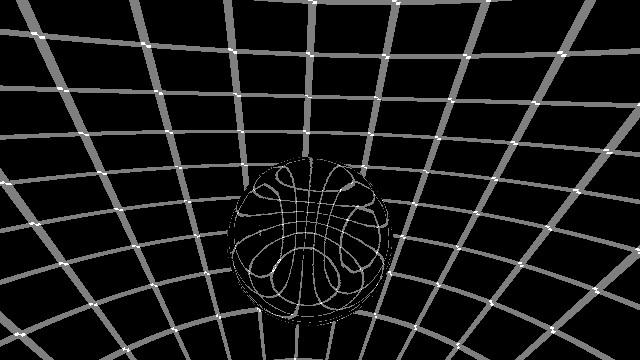• @ nabr nice code! after replacing refDir as you suggested, there aren't any seams no longer. for a quick test, i loaded this simple meshterrain :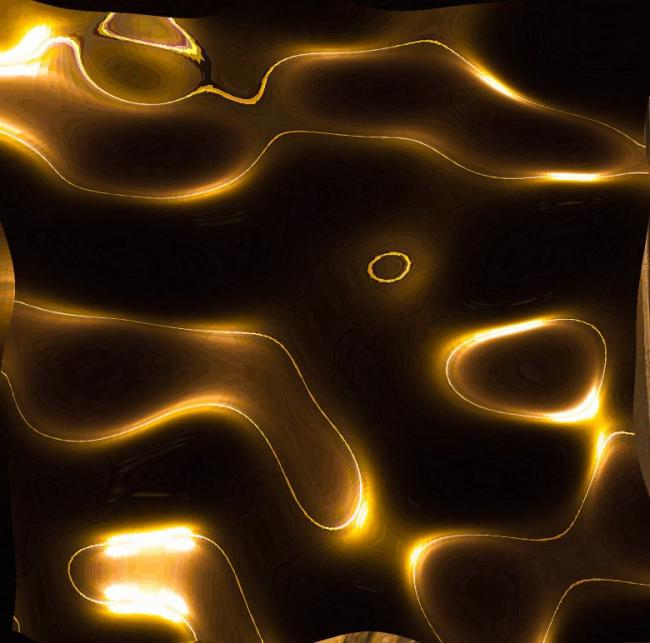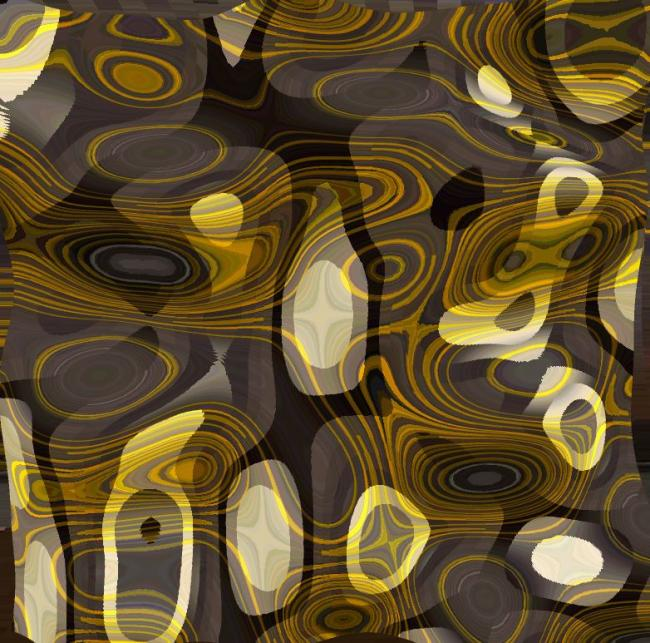• edited May 2017
``````//
//fixed rotation

PMatrix3D rotY=new PMatrix3D();
PMatrix3D model = new PMatrix3D();
PMatrix3D modelview = new PMatrix3D();

float x =0;

PShape s;

void setup(){
size(856,480,P3D);

//for debug leave it here
//noLights();noStroke();noSmooth();

modelview=((PGraphicsOpenGL)g).modelview;

new String[]{"#version 150  \n"
+ "in vec4 position; "
+ "uniform mat4 Ry,view;"
+ "out vec4 refDir;"
+ "void main() {"
+ "gl_Position = vec4(position.xy,.0, 1.);"

//for reasons for taste i apply the rotation matrix first
+ "refDir =Ry*view*vec4(position.xy,1.,0);"
+ "}"
}, new String[] {"#version 150  \n"
+ "in vec4 refDir;"
+ "uniform sampler2D envmap;"
+ "out vec4 fragColor;"
+ "const float PI ="+(double)PI+";"
+ "void main () {"
//reindelsoftware.com/Documents/Mapping/Mapping.html
+ "fragColor = texture(envmap,"
+                "vec2(.5+atan(refDir.z,refDir.x)/(2.0*PI),"
+                        "acos(refDir.y/length(refDir))/PI));"
+ "}"
}){

//mode: linear
((PGraphicsOpenGL)g).textureSampling(2);

//somewhere else i run this minimal clear background

//somewhere else i run this minimal clear background
//fill(x);
//rect(x,x,width,height);
////draw grid
//stroke(254);
//while(x<width){
//x+=20.01f;
//line(x,0,x,height);
//line(0,x,width,x);
//} //x=0;

//this.set("envmap",get() );

//for debug leave it here
//this.set("modelview",((PGraphicsOpenGL)g).modelview);

return this;
}
}.run();
new String[]{ "#version 150  \n"
+  "in vec3 position,normal;"
+ "uniform mat4 modelviewInv,view,projection;"
+ "uniform float time;"
+ "out vec3 refDir;"
+ "void main () {"
+ "vec4 camPos = view*vec4(position, 1);"
//+ "vec3 eye = normalize(position.xyz-modelviewInv.xyz/modelviewInv.w);"

//changed
+ "refDir =  -normalize(normal);"

//+ "camPos.z*=clamp(sin(time)*.5+.5,0,.5);"
+ "gl_Position = projection*camPos;"
+ "}"
},new String[]{"#version 150  \n"
+  "in vec3 refDir;"
+ "uniform sampler2D envmap;"
+ "out vec4 fragColor;"
+ "const float PI ="+(double)PI+";"
+ "void main () {"
/* + "fragColor = texture(envmap,"
+                "vec2(.5+atan(refDir.z,refDir.x)/(2.0*PI),"
+                        "acos(refDir.y/length(refDir))/PI));"*/
//mvps.org/directx/articles/spheremap.htm

//+ "fragColor = texture(envmap,vec2( .5+asin(refDir.x/PI) ,.5+ asin(refDir.y/PI) ));"

//thinking of a sphere ...
+ "fragColor = texture(envmap,.5+vec2(refDir/PI).xy);"

+"}"
}){
this.set("modelviewInv",((PGraphicsOpenGL)g).modelviewInv);

//for debug leave it here
//this.set("modelview",   ((PGraphicsOpenGL)g).modelview);

this.set("projection",  ((PGraphicsOpenGL)g).projection);
return this;
}
}.run();

//with both options off/on you can see their some weird things happens

//for debug leave it here
//hint(DISABLE_OPTIMIZED_STROKE);

//for debug leave it here
//wireframe Mode
noStroke();
}
float t=0;
void draw(){

t=millis()*.0005f;

camera();

//rotate Camera
//rotateX(sin(t));rotateZ(cos(t)*.5+.5);

//load the Identity matrix first PMatrix3D() returns the Identity matrix
//is far as i understand it.

//reset the stack
rotY.reset();

//unnecessary code for rotation or push and popMatrix
//rotY.apply(cos(t), 0, sin(t), 0, 0, 1, 0, 0, -sin(t), 0, cos(t), 0, 0, 0, 0, 1);

//bug: apply view matrix with processing rotation in both shaders i dont really need to rotate a second time

rotY.apply(
1, 0, 0, 0,
0, 1, 0, 0,
0 ,0, 1, 0,
0, 0, 0, 1);

//set time

//no depth testing

//for debug leave it here
background(0);

rect(0, 0, width, height);

//translate(width/2, height/2, 0);sphere(120);

//push and popmatrix will close a matix transformation if needed
//pushMatrix();

translate(width/2, height/2+50);

rotateY(t);
scale(50);
shape(s);

//after we apply the transformation to the sphere we update the matrix
//and going back to the begining of the draw loop ->camera

//load the modelview from stack first then update it

//reset the stack
model.reset();
model.apply(modelview.m00, modelview.m10, modelview.m20, modelview.m30,
modelview.m01, modelview.m11, modelview.m21, modelview.m31,
modelview.m02, modelview.m12, modelview.m22, modelview.m32,
modelview.m03, modelview.m13, modelview.m23, modelview.m33);

//popMatrix();

//for debug leave it here
//if(keyPressed||mousePressed)exit();
}
``````
• edited May 2017

@HBo yeah unnecessary code for rotation

you dont really need a *.obj for a simple model if you got shaders :)

http://www.ozone3d.net/tutorials/vertex_displacement_mapping.php

• @nabr yes i know the power of shaders. but i also know, how much more it still takes for me, until i see something on screen or even understand, what i'm doing. i'll deal with that, when i get in trouble with the framerate

• edited May 2017

Yes, i have a copy paste bug like everywhere

this is wrong

`rotateZ(cos(t)*.5+.5);`

explanation

``````PShader bgShader;

PMatrix3D rotY=new PMatrix3D();
PMatrix3D model = new PMatrix3D();
PMatrix3D modelview = new PMatrix3D();

float x =0;
void setup() {
size(856, 480, P3D);
modelview=((PGraphicsOpenGL)g).modelview;

noStroke();

//hint(DISABLE_OPTIMIZED_STROKE);

new String[]{"#version 150  \n"
+ "in vec4 position; "
+ "uniform mat4 view;"
+ "out vec4 refDir;"
+ "void main() {"
+ "gl_Position = vec4(position.xy,.0, 1.);"
+ "refDir =view*vec4(position.xy,1.,0);"
+ "}"
}, new String[] {"#version 150  \n"
+ "in vec4 refDir;"
+ "uniform sampler2D envmap;"
+ "out vec4 fragColor;"
+ "const float PI ="+(double)PI+";"
+ "void main () {"
//reindelsoftware.com/Documents/Mapping/Mapping.html
+ "fragColor = texture(envmap,"
+                "vec2(.5+atan(refDir.z,refDir.x)/(2.0*PI),"
+                        "acos(refDir.y/length(refDir))/PI));"
+ "}"
}){
((PGraphicsOpenGL)g).textureSampling(2);
fill(x);
rect(x, x, width, height);
//draw grid
stroke(254);
while (x<width) {
line( x+=20.01f, 0, x, height);
line(0, x, width, x);
}//x=0;
this.set("envmap",get());
return this;
}
}.run();
}

double timed=0;
float  timef=0;

void draw() {

//yes, i get used to coding everything by myself mostly in glsl c like language
//opengl runs with  glRotatef and glPerspective and performs in degrees

//and then i use java/processing rotateY and performs in radians : )
//tutorialspoint.com/java/lang/math_cos.htm
//so some things are gonna break.

//cos is going from -1 to 1
//for example +.5*5. is a range -1.+.5*5.=1.5 to 1.+.5*5.=3.5
//i put up some nummbers here, you have to use the calc basicly i do tests for different numbers

//and it fails at some "nummbers" like 0.84 but not at 0.52 ~PI/6
//processing.org/reference/rotateY_.html

timed=millis()*.001d;

//Proceesig way
timef=millis()*.001f;

//just to make sure i did nothing wrong using Java Math
double a =Math.cos(timed)+.5d*5.d;
float tmpd =(float)(a);
//rotateY(tmpd);println(tmpd);

float tmpf = cos(timef)+.5f*5.f;
//rotateY(tmpf);println(tmpf);

rotateY(cos(timef)*.5+.5);
//println timef will give me timef*timef =~something
//so its nessasary to create a tmp variable
//or reset the Matrix via push and then popMatrix()
//or divide by PI might help
//or just don't do it this way

translate(width/2, height/2, 0);
sphere(120);

model.reset();
model.apply(modelview.m00, modelview.m10, modelview.m20, modelview.m30, modelview.m01, modelview.m11, modelview.m21, modelview.m31, modelview.m02, modelview.m12, modelview.m22, modelview.m32, modelview.m03, modelview.m13, modelview.m23, modelview.m33);
}
``````

EDIT no i was wrong (maybe) I have to rotate the camera! camera(sin(),cos()..... ) !

• edited June 2017

@HBo i hope, it's okay i use your test_terrain.obj on my >github < it's public. When not: PN me and i will take it down.

• @ nabr no problem :)